Looking for revision notes that are specific to the exam board you are studying? If so, click the links below to view our condensed, easy-to-understand revision notes for each exam board, practice exam question booklets, mindmap visual aids, interactive quizzes, PowerPoint presentations and a library of past papers directly from the exam boards.

# Shapes of Molecules

## Key Information & Summary

• The Molecular orbital theory is a technique for determining the molecular structure
• In molecular orbital theory, we postulate that the combination of atomic orbitals on different atoms forms molecular orbitals (MOs)
• These orbitals may be pure atomic orbitals or hybridized atomic orbitals of individual atoms.
• We then use hybridization to help account for the geometry of a molecule.
• A molecular orbital is represented by a function whose square describes the distribution of probability relative to the position of the electron.
• A Bonding MO corresponds to the sum of the wave functions of the two orbitals, has low energy, bring to constructive interaction (to bind).
• An antibonding MO corresponds to the subtraction of the wave functions of the two orbitals, has high energy, bring to destructive interaction .
• Nonbonding MOs are the result of no interaction between atomic orbitals because of lack of compatible symmetries.

In valence bond theory, we postulate that bonds result from the sharing of electrons in overlapping orbitals of different atoms. These orbitals may be pure atomic orbitals or hybridized atomic orbitals of individual atoms. We describe electrons in overlapping orbitals of different atoms as being localized in the bonds between the two atoms involved. We then use hybridization to help account for the geometry of a molecule.

In molecular orbital theory, we postulate that the combination of atomic orbitals on different atoms forms molecular orbitals (MOs), so that electrons in them belong to the molecule as a whole.

Valence bond and molecular orbital theories are complementary descriptions of chemical bonding. They have strengths and weaknesses, so they are complementary.

In chemistry, particularly in quantum chemistry, a molecular orbital is the spatial distribution of electrons in a molecule. Introduced by Friedrich Hund and Robert S. Mulliken in 1927 and 1928, a molecular orbital is represented by a function whose square describes the distribution of probability relative to the position of the electron.

This wave function is obtained from the wave equation that describes the entire molecule, which in general is not easy to solve: this problem is solved by an approximation consisting in writing the molecular orbital as a linear combination of the orbitals atomic of individual atoms. This approximation is described by the theory of molecular orbitals.

The Molecular orbital theory is a technique for determining the molecular structure in which electrons are not assigned to particular chemical bonds, but are treated as objects that move under the influence of nuclei within the entire molecule.

The total wave function of the electrons ψ is written as a linear combination of atomic orbitals. Thus a molecular orbital can be visualized as the product of the overlapping of two atomic orbitals.

Atomic orbitals: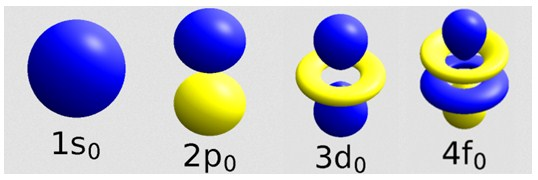When the overlap occurs along the two nuclei joining, the molecular orbital takes the name of σ (sigma);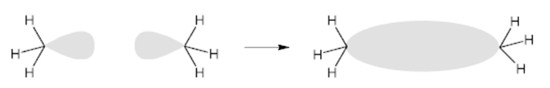when the superposition occurs perpendicular to the axis that unites the two nuclei, or above and below the same, the molecular orbital takes the name of π (greek pi ).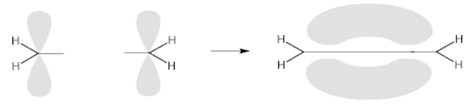A wave function that describes the motion of an electron around multiple nuclei in the presence of other electrons is extremely complex. So an approximations is used by representing them as a linear combination of atomic orbitals from which they they derive by overlap (LCAO method, from linear combination of atomic orbitals).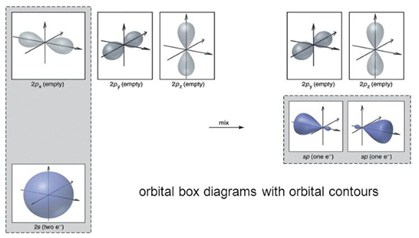According to the LCAO method, the overlapping of two atomic orbitals produces two molecular orbitals. The low energy one, called bonding orbital, corresponds to the sum of the wave functions of the two orbitals. The high-energy one, called an antibonding orbital, corresponds to the subtraction of the wave functions of the two orbitals. The nonbonding orbitals exists too. They are not the result of overlapping, they are irrelevant to the energy stability of the molecular structure but they influence the chemical reactivity.

Summarizing:

Bonding MOs:

Bonding interactions between atomic orbitals are constructive interactions.

Bonding MOs are lower in energy than the atomic orbitals that combine to produce them.

Antibonding MOs:

Antibonding interactions between atomic orbitals are destructive interactions, with a nodal plane where the wavefunction of the antibonding orbital is zero between the two interacting atoms.

Antibonding MOs are higher in energy than the atomic orbitals that combine to produce them.

Nonbonding MOs:

Nonbonding MOs are the result of no interaction between atomic orbitals because of lack of compatible symmetries.

Nonbonding MOs will have the same energy as the atomic orbitals of one of the atoms in the molecule.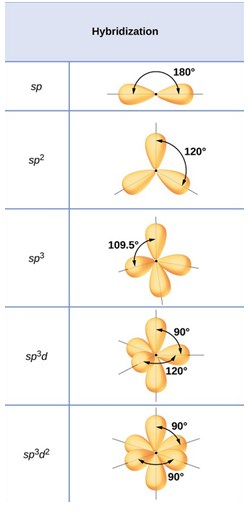Example

Let's apply as an example the LCAO method to obtain the molecular orbitals of a relatively simple structure, such as that of hydrogen fluoride (chemical symbol HF). First of all, we need to know the electronic configurations of the atoms that make up the molecule:

for hydrogen (H): 1s1

for fluorine (F): 1s2 2s2 2p2x 2p2y 2p1z

Now let's analyze the possible linear combinations, i.e. the combinations of two different orbitals having comparable energy and the same spatial orientation. Only the 1s orbital is superimposable of hydrogen with the 2s and 2px orbital axes (with x binding nuclear axis).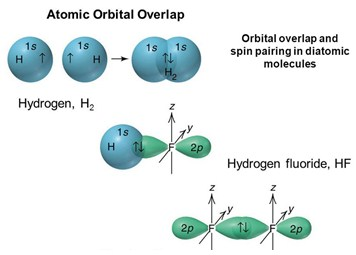Moreover, the 2py and 2pz fluoride orbitals remain unaltered as they show π symmetry and there are no hydrogen orbitals that can combine with these; they will originate a molecular orbital, not binding π. Eight total electrons (1 of H + 7 of F) assume for the HF molecule the configuration 1σ2 2σ2 1π4, with 1σ molecular binding orbital, 2σ essentially non-binding orbital and the non-binding 1π orbital. There is also an empty 3σ molecular orbital of an anti-binding type.

By dividing by two the result of the difference between the electrons contained in the binding orbitals and those contained in the anti-binders, we obtain the binding order.